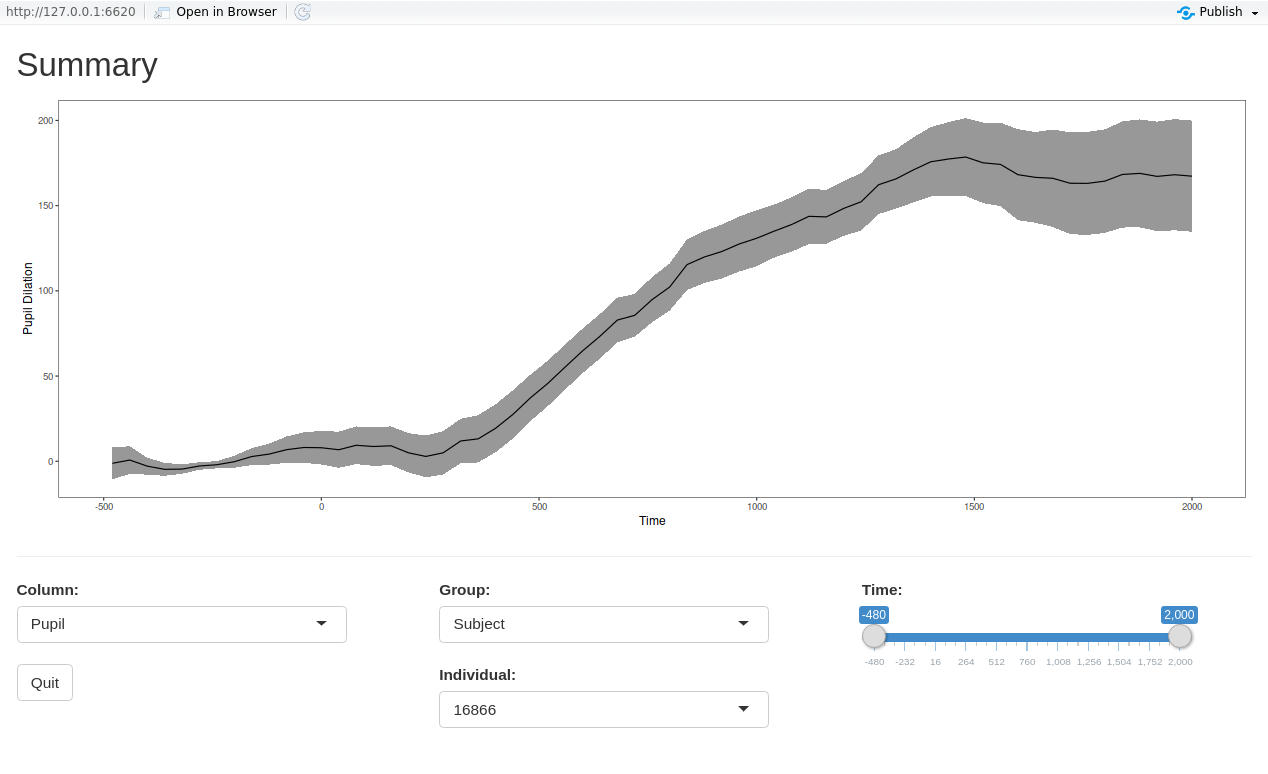Plotting Pupil Size Data Processed with PupilPre

Plotting the data

It is often desirable to visualize the preprocessed data, either as a grand average or by condition. In some cases it is even necessary to visualize the trend in the data over a continuous predictor. So, the functions ppl_plot_avg and ppl_plot_avg_contour provide straightforward plotting options for such cases. These functions internally calculate the average(s) and plot the results. The plotting is powered by ggplot2, so further customization (plot titles, custom themes, etc.) is still possible. For more information about ggplot2, please refer to its reference manual and extensive documentation.

Averaged data

Using the function ppl_plot_avg, it is possible to plot the overall average of the data. In Column simply put the column containing the pupil size data

ppl_plot_avg(data = dat, xlim = c(0, 2000), Column = "Pupil",
Condition1 = NULL, Condition2 = NULL, Cond1Labels = NA,
Cond2Labels = NA, ErrorBar = TRUE, PupilPreTheme = TRUE)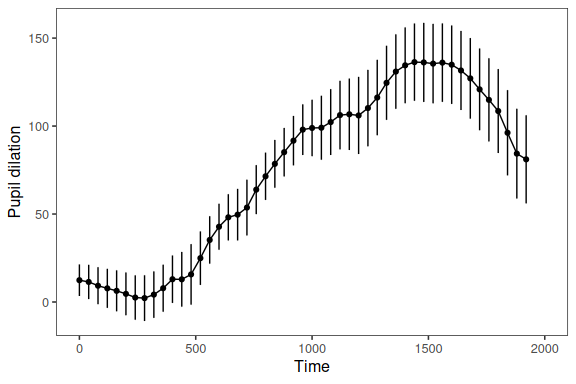To add a title to the plot, simply add the title function from ggplot2.

ppl_plot_avg(data = dat, xlim = c(0, 2000), Column = "Pupil",
Condition1 = NULL, Condition2 = NULL, Cond1Labels = NA,
Cond2Labels = NA, ErrorBar = TRUE, PupilPreTheme = TRUE) +
ggtitle("Averaged Data")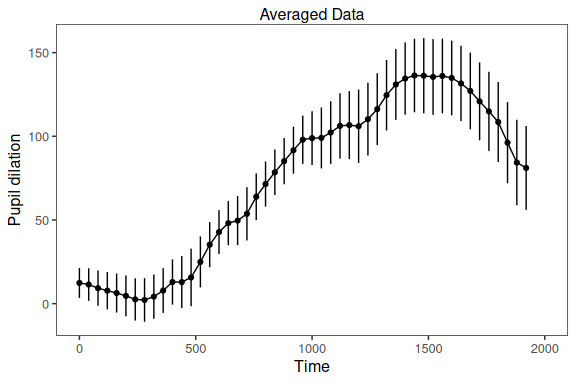To customize the appearance of a plot (e.g., font, size, color, margins, etc.), the PupilPreTheme parameter can be set to FALSE, which reverts to the default theming in gglpot2. In this way, the user can apply a custom theme to the plot. Detailed information about creating themes can be found at ggplot2 Theme Vignette. For the purpose of illustration, the default ggplot2 theme has been applied, with the axis text elements increased in size.

ppl_plot_avg(data = dat, xlim = c(0, 2000), Column = "Pupil",
Condition1 = NULL, Condition2 = NULL, Cond1Labels = NA, Cond2Labels = NA,
ErrorBar = TRUE, PupilPreTheme = FALSE) +
theme(axis.text = element_text(size = 15))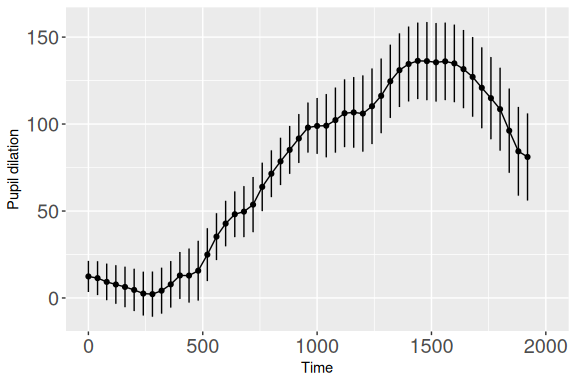Conditional averages

The function ppl_plot_avg can also be used to plot averages for different conditions, based on a factor variable in the data. If the current factor level labels are not suitable for plotting, specify new labels using a list in Cond1Labels. This will add curves for all levels in a single plot.

ppl_plot_avg(data = dat, xlim = c(0, 2000), Column = "Pupil",
Condition1 = "talker", Condition2 = NULL,
Cond1Labels = c(CH1 = "Chinese 1", CH8 = "Chinese 3",
CH9 = "Chinese 2", CH2 = "Chinese 4", EN3 = "English 1"),
Cond2Labels = NA, ErrorBar = TRUE, PupilPreTheme = TRUE)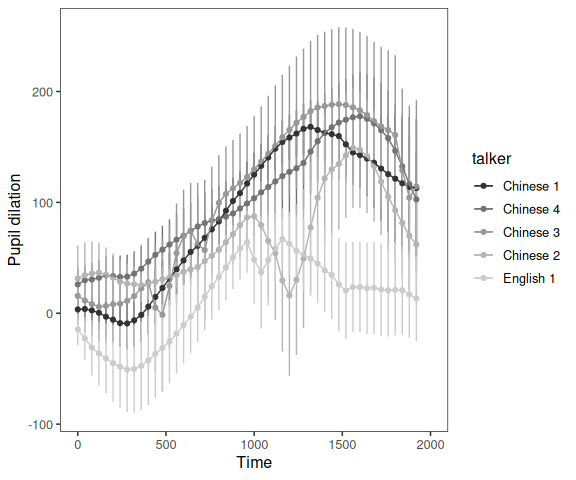For a 2x2 design, it is possible to specify both conditions. This will create the interaction of the two conditions and plot all combinations in a single plot

ppl_plot_avg(data = dat, xlim = c(0, 2000), Column = "Pupil",
Condition1 = "talker", Condition2 = "ExpLev",
Cond1Labels = c(CH1 = "Chinese 1", CH8 = "Chinese 3",
CH9 = "Chinese 2", CH2 = "Chinese 4", EN3 = "English 1"),
Cond2Labels = c(H = "High Exp", L = "Low Exp"),
ErrorBar = TRUE, PupilPreTheme = TRUE)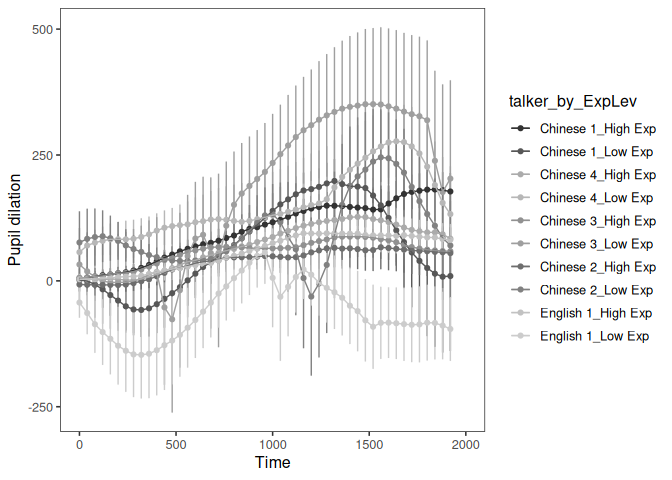Error bars and Error bands

Error around the means can be plotted in two ways, either as error bars or as error bands. Note that with the included theme, the colors and shapes change for error bands to maximize readablity.

gridExtra::grid.arrange(

ppl_plot_avg(data = dat, xlim = c(0, 2000), Column = "Pupil",
Condition1 = NULL, Condition2 = NULL, Cond1Labels = NA,
Cond2Labels = NA, ErrorBar = TRUE, PupilPreTheme = TRUE),

ppl_plot_avg(data = dat, xlim = c(0, 2000), Column = "Pupil",
Condition1 = NULL, Condition2 = NULL, Cond1Labels = NA,
Cond2Labels = NA, ErrorBar = FALSE,
ErrorBand = TRUE, PupilPreTheme = TRUE),

nrow=2, ncol=1)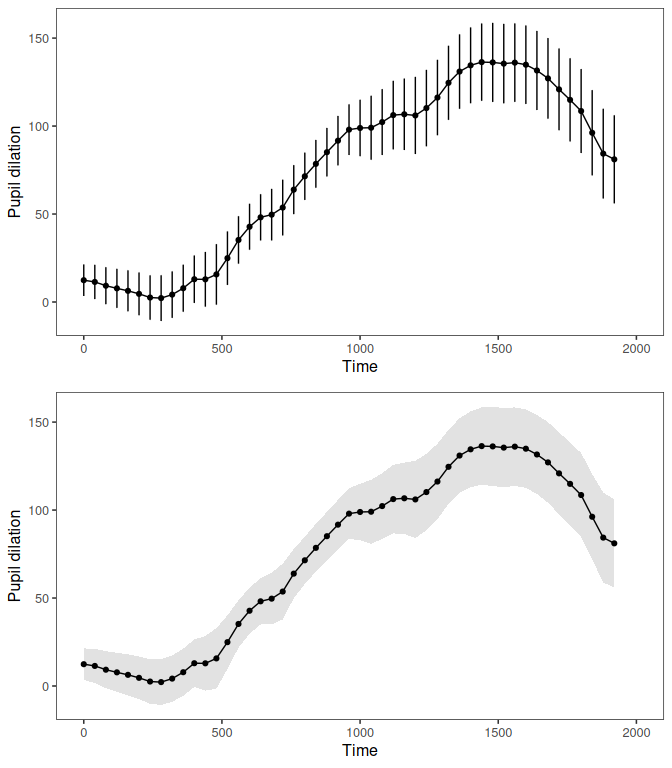Confidence intervals (pointwise/simultaneous)

Rather than having standard error plotted, it is possible to have confidence intervals added to the graph. Confidence intervals can be plotted either as bars or bands and controlled using ErrorType and ConfLev. ErrorType should be set to “CI” for confidence intervals, and ConfLev set to the desired levels of confidence (by default 95). An important option is the type of desired interval, either pointwise or simultaneous, using CItype. Pointwise CIs use the confidence level provided for each interval plotted. Simultaneous CIs are, on the other hand, corrected for multiple intervals using the Bonferroni method. Underlyingly this correction is done using the number of data points along the x-axis (Time).

gridExtra::grid.arrange(

ppl_plot_avg(data = dat, xlim = c(0, 2000), Column = "Pupil",
Condition1 = NULL, Condition2 = NULL, Cond1Labels = NA,
Cond2Labels = NA, ErrorBar = FALSE, ErrorBand = TRUE,
ErrorType = "CI", ConfLev = 95, CItype = "pointwise",
PupilPreTheme = TRUE),

ppl_plot_avg(data = dat, xlim = c(0, 2000), Column = "Pupil",
Condition1 = NULL, Condition2 = NULL, Cond1Labels = NA,
Cond2Labels = NA, ErrorBar = FALSE, ErrorBand = TRUE,
ErrorType = "CI", ConfLev = 95, CItype = "simultaneous",
PupilPreTheme = TRUE),

nrow=2, ncol=1)
## Grand average calculated using Event means.
## Plot created with pointwise confindence intervals (set to 95%).
## Grand average calculated using Event means.
## Plot created with simultaneous confindence intervals (adjusted to 99.95% using the Bonferroni method).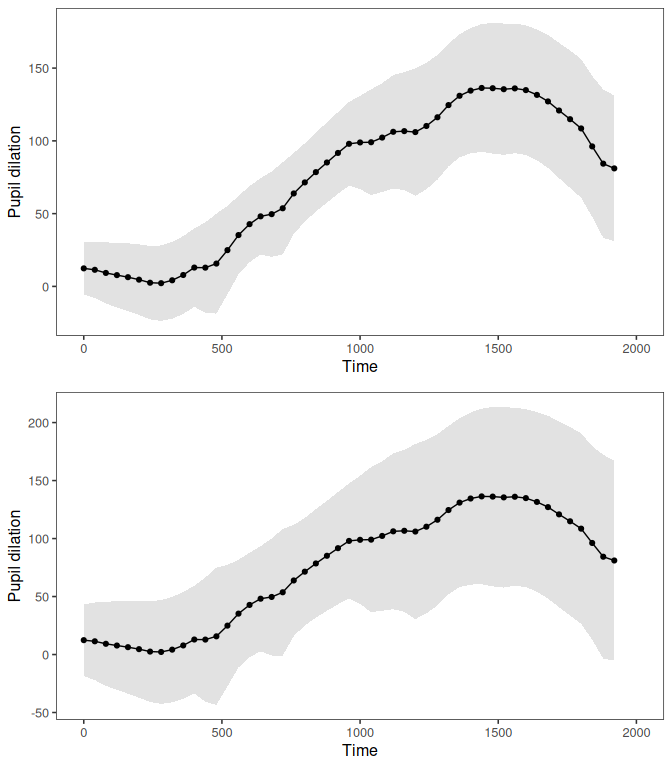Calculation of averages - Mean vs. Grand mean

By default, the plotting function calculates the overall mean in the data set by time. However, by changing the input to the parameter Averaging, it is possible to supply a column name for which grand mean (mean of means) can be calculated (e.g., “Subject”). Note that for balanced data, the resultant mean will be the same, but the size of the error bars/bands will change. For example, given the small number of participants in this data set, the error bars become much wider.

ppl_plot_avg(data = dat, xlim = c(0, 2000), Column = "Pupil",
Condition1 = NULL, Condition2 = NULL, Cond1Labels = NA,
Cond2Labels = NA, Averaging = "Subject", ErrorBar = TRUE,
ErrorType = "SE", PupilPreTheme = TRUE)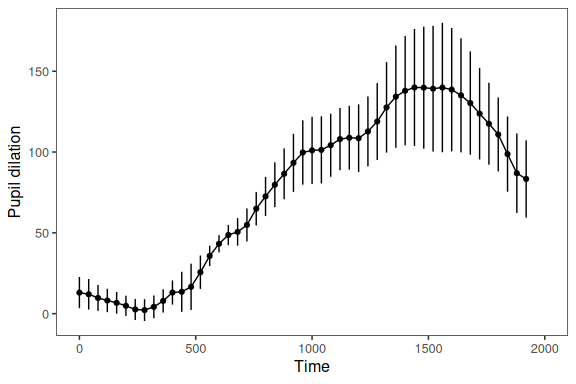Difference plots

The function ppl_plot_avg_cdiff can also be used to plot the average difference between two conditions.

ppl_plot_avg_cdiff(data = dat, Column = "Pupil",
Condition = list(talker = c("EN3", "CH1")),
ErrorBar = TRUE, PupilPreTheme = TRUE)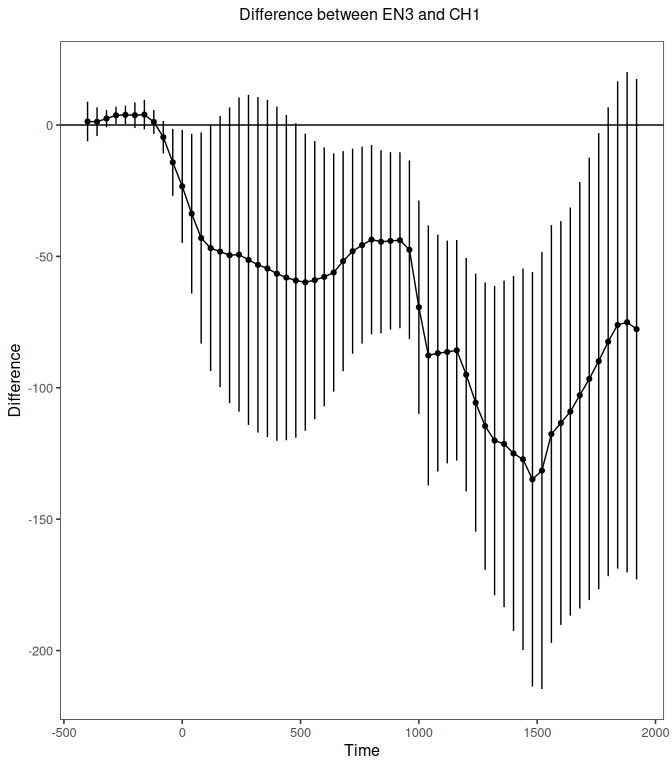Error bands and confidence intervals (both pointwise and simultaneous) work the same way as in the ppl_plot_avg function. With regard to averaging, please note that the function will check that both levels of the condition occur for each level of the column provided in Averaging (e.g., Subject or Item), as this is necessary to calculate the difference based on subject or item means. If this is not the case, the function will stop. If you would still like to produce the plot based on the difference between sample means of the levels, you can set Averaging = NULL.

Conditional contour surface

In some cases, studies have not employed a factorial design; rather they aim to investigate continuous variables. Therefore, using the function ppl_plot_avg_contour it is also possible to create a contour plot representing the looks to one interest area as a surface over the continuous variable and Time. This function calculates the average time series at each value of the continuous variable and applies a smooth (utilizing the function gam in the package mgcv) over the surface. The function then plots the result as a contour plot. Here, the example plots pupil dilation as a function of Trial and Time.

ppl_plot_avg_contour(data = dat, Column = "Pupil", Var = "TRIAL_INDEX",
VarLabel = "Trial", xlim = c(0,2000), PupilPreTheme = TRUE,
Colors = c("gray20", "gray90"))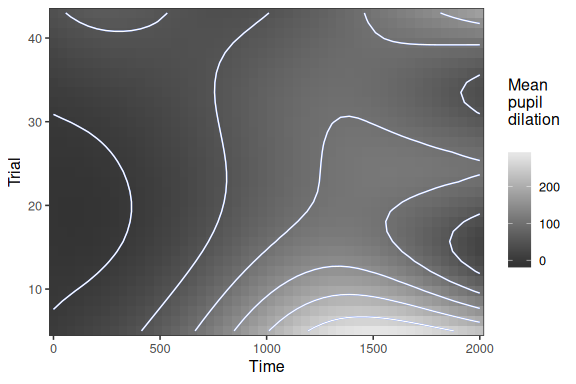It is possible to change the contour colors and add a title. ggplot2 accepts predefined palette colors, RGB, hexadecimal, among others.

ppl_plot_avg_contour(data = dat, Column = "Pupil", Var = "TRIAL_INDEX",
VarLabel = "Trial", xlim = c(0,2000), PupilPreTheme = TRUE,
Colors = c("red", "green")) + ggtitle("Pupil dilation")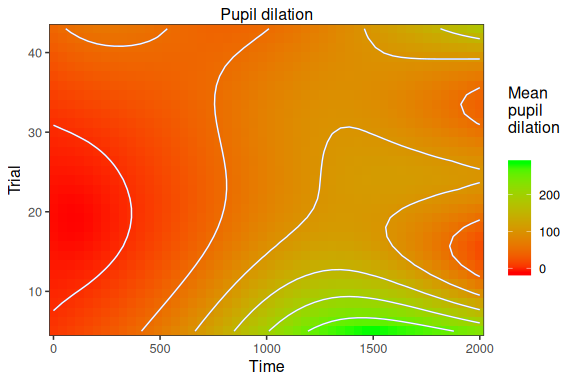Averaging works the same way as in the ppl_plot_avg function.

Extracting plotting data

Because the plotting functions are based on ggplot2 it is possible to easily extract the data used to create the plot.
This is useful if you desire a highly custom plot, but would like to use the averages and error calculations produced by the plotting function.

To extract the data, first save the plot to an object in your workspace. This will create a list object containing the data.

plt <- ppl_plot_avg(data = dat, xlim = c(0, 2000), Column = "Pupil",
Condition1 = NULL, Condition2 = NULL, Cond1Labels = NA,
Cond2Labels = NA, Averaging = "Subject", ErrorBar = TRUE,
ErrorType = "SE", PupilPreTheme = TRUE)

The data can then be accessed by extracting the dataframe from the list.

df <- plt\$data
IA Time mean n se ci error_lower error_upper
Pupil 0 13.025186 5 9.653499 82.68204 3.3716870 22.67869
Pupil 40 12.017160 5 9.448592 80.92701 2.5685677 21.46575
Pupil 80 9.722882 5 8.012367 68.62577 1.7105146 17.73525
Pupil 120 8.156133 5 7.213615 61.78447 0.9425180 15.36975
Pupil 160 6.698177 5 6.696125 57.35219 0.0020513 13.39430
Pupil 200 4.851130 5 6.254209 53.56718 -1.4030783 11.10534

Event plotting

Often it is helpful to view the time course of events at a particular stage of processing. The function plot_events will produce event plots which are grouped in a meaningful way. The argument Grouping is supplied with the column to serve as the grouping factor. The resulting plots will be placed in a directory supplied in path. If the directory does not already exist, it will be created. The argument Device indicates the plotting device (e.g., pdf, jpeg, etc.). The function underlyingly relies on ggsave, so agruments specific to ggsave (e.g., width, height, etc.) can be passed.

plot_events(pupiltest4, Column = "Pupil", Device = "pdf", Grouping = "Subject", path = "Auto", Nrow = 2, Ncol = 3, width = 11, height = 8.5)

Interactive/Utility plotting apps

Shiny app plots for data inspection

There are two functions which provide diagnostic Shiny apps for inspecting the data: plot_compare_app and plot_summary_app. These are interactive and allow the user to inspect specific events, subjects and items.

The function plot_compare_app allows the user to view specific events comparing the data contained in the columns Pupil and Pupil_previous. For this the user provides the desired time window.

plot_compare_app(dat)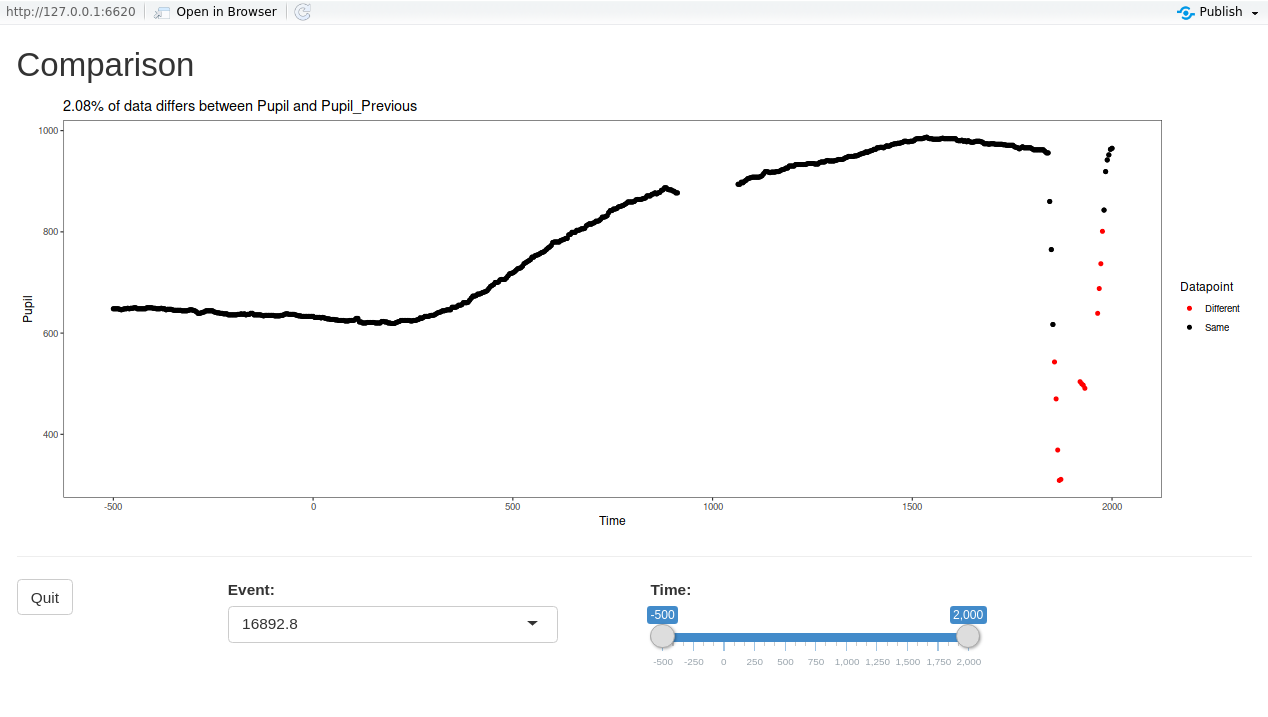The function plot_summary_app allows the user to view by-subject or by-item averages. For this the user selects the column, group, and time window.

plot_summary_app(dat)